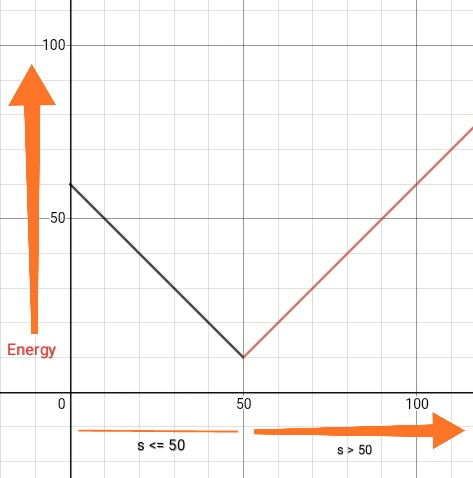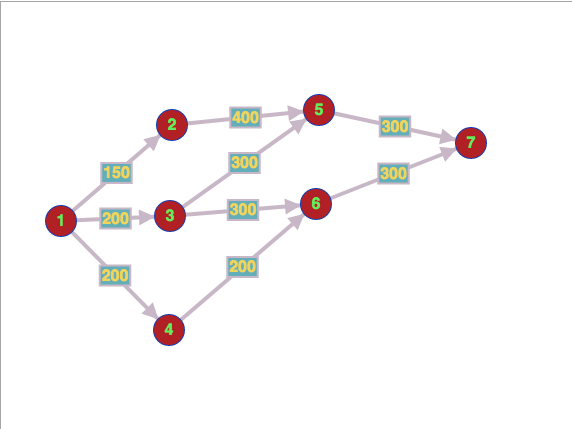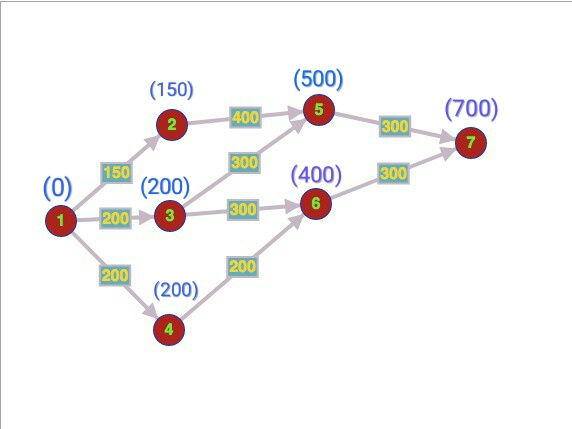# ZIO05005 - Editorial

Editorialist : Sudheera Y S

Prerequisites : graphs , DP

Problem statement :

There are some flat areas and some slopes connecting two flat areas . A slope area has some length d and some maximum speed s in which we can go in that slope with that maximum speed. Going on the slope takes some energy as defined below :

If s <= 50 → energy e = d*(60 - s )

If s > 50 → energy e = d*(s - 40 )

Everytime you use some slope to get to the other flat area , you add the energy required to go in that slope to your total energy. You are in flat 1 and you want to reach flat area n , find the minimum total energy from which we can reach flat n

Idea :

First for each slope , we find the minimum energy required if we travel on this slope and then once we do this for all the slopes , as the graph is topologically sorted , we do DP to find the minimum energy required to reach each flat area and then we would find our answerNumber of flat areas : 7

Number of slopes : 9

Slopes :

No. → 1 2 3 4 5 6 7 8 9
Fro → 1 1 1 2 3 3 4 5 6
To → 2 3 4 5 5 6 6 7 7
s → 60 40 20 40 60 30 20 30 30
d → 15 10 5 20 30 10 5 10 10
e →

NOTE : Here s is maximum speed , so we can go in any speed between 1 and s both inclusive

Let us denote E(x,y) as the energy required to use the slope which connects flat areas x and y

E( 1,2 ) :

Here , s > 50 , therefore energy required is (s - 40)d = 2015 = 300 if we go in 60 speed

Now what if we go at 51 speed ?

Here also , s > 50 , therefore energy required is (s - 40 )d = 1115 = 165 if we go in 51 speed

But what if we go at 50 speed ?

Here , s <= 50 , therefore energy required is (60 - s)d = 1015 = 150 if we go in 50 speed which is lesser than both of the above

What if we go at a speed less than 50 ?

Clearly , 60 - s would increase , hence increasing the energy required

Therefore, the formula for finding the minimum energy required is

Minimum energy = d * ( 60 - min( 50 , s ) )

Here we are doing min ( 50 , s ) because if s > 50 , then it takes as 50 making the energy minimum

Let us plot it in graph and see it graphically that taking 50 if s > 50 is better :Here we see that , taking s = 50 ( when s > 50 ) is better than taking s > 50 or taking s < 50

So now , let us calculate the energy required for all the slopes :

E(1, 2) = d * ( 60 - min( 50 , s )) = 15*10 = 150

E(1, 3) = d * ( 60 - min( 50 , s )) = 10*20 = 200

E(1, 4) = d * ( 60 - min( 50 , s )) = 5*40 = 200

E(2, 5) = d * ( 60 - min( 50 , s )) = 20*20 = 400

E(3, 5) = d * ( 60 - min( 50 , s )) = 30*10 = 300

E(3, 6) = d * ( 60 - min( 50 , s )) = 10*30 = 300

E(4, 6) = d * ( 60 - min( 50 , s )) = 5*40 = 200

E(5, 7) = d * ( 60 - min( 50 , s )) = 10*30 = 300

E(6, 7) = d * ( 60 - min( 50 , s )) = 10*30 = 300

The table would look like this :

No. → 1 2 3 4 5 6 7 8 9
From → 1 1 1 2 3 3 4 5 6
To → 2 3 4 5 5 6 6 7 7
s → 60 40 20 40 60 30 20 30 30
d → 15 10 5 20 30 10 5 10 10
e → 150 200 200 400 300 300 200 300 300

The graph would look something like this :Let us do DP now to find our answer :

Before doing DP , we have to know if dp works here ,

The answer is ‘Yes’ as the graph is topologically sorted and there are no cycles ( the graph is DAG i.e Directed Acyclic Graph )

So now , after making sure that DP works , let us see how to do DP :

Dp[ i ] = minimum energy required to reach the i’th flat area

Base case : dp[ 1 ] = 0 because we start at the first flat area and reaching the first flat area requires no energy , therefore this is the base case

Relation : dp[ i ] = min ( dp[ j ] + E ( i,j ) ) for all j from which we can come to i’th flat area using some slope

Answer : dp[ n ] because we have to find the minimum energy required to reach the n’th flat area

So now , let us start filling the dp table :

Base case : dp[ 1 ] = 0

Relation :

Dp[ 2 ] = dp [ 1 ] + E(1,2) = 0 + 150 = 150 ( as we can only come from 1)

Dp[ 3 ] = dp [ 1 ] + E(1,3) = 0 + 200 = 200 ( as we can only come from 1)

Dp[ 4 ] = dp [ 1 ] + E(1,4) = 0 + 200 = 200 ( as we can only come from 1)

Dp[ 5 ] = min ( dp[ 2 ] + 400 , dp[ 3 ] + 300 ) = min ( 700 , 800 ) = 700

Dp[ 6 ] = min ( dp[ 3 ] + 300 , dp[ 4 ] + 200 ) = min ( 500 , 400 ) = 400

Dp[ 6 ] = min ( dp[ 5 ] + 300 , dp[ 6 ] + 300 ) = min ( 1000 , 700 ) = 700

Answer : dp[ 7 ] = 700

Dp values for all the points :Number of flat areas : 9

Number of slopes : 12

Slopes :

Now , let us calculate the energy for all the slopes :

E(1, 2) = d * ( 60 - min( 50 , s )) = 15*10 = 150

E(1, 3) = d * ( 60 - min( 50 , s )) = 25*20 = 500

E(2, 4) = d * ( 60 - min( 50 , s )) = 30*20 = 600

E(2, 5) = d * ( 60 - min( 50 , s )) = 25*10 = 250

E(3, 5) = d * ( 60 - min( 50 , s )) = 20*10 = 200

E(3, 6) = d * ( 60 - min( 50 , s )) = 10*20 = 200

E(4, 7) = d * ( 60 - min( 50 , s )) = 10*30 = 300

E(5, 7) = d * ( 60 - min( 50 , s )) = 10*10 = 100

E(5, 8) = d * ( 60 - min( 50 , s )) = 5*20 = 100

E(6, 8) = d * ( 60 - min( 50 , s )) = 20*20 = 400

E(7, 9) = d * ( 60 - min( 50 , s )) = 10*20 = 200

E(8, 9) = d * ( 60 - min( 50 , s )) = 20*40 = 800

The table would look like :

No. → 1 2 3 4 5 6 7 8 9 10 11 12
From → 1 1 2 2 3 3 4 5 5 6 7 8
To → 2 3 4 5 5 6 7 7 8 8 9 9
s → 50 40 40 50 50 40 30 60 40 40 40 20
d → 15 25 30 25 20 10 10 10 5 20 10 20
E → 150 500 600 250 200 200 300 100 100 400 200 800

The graph would look something like this :

So now , let us start filling the dp table :

Base case : dp[ 1 ] = 0

Relation :

Dp[ 2 ] = dp[ 1 ] + 150 = 150 ( as we can only come from 1)

DP[ 3 ] = dp[ 1 ] + 500 = 500 ( as we can only come from 1)

Dp[ 4 ] = dp[ 2 ] + 600 = 750 ( as we can only come from 2)

Dp[ 5 ] = min ( dp[ 2 ] + 250 , dp[ 3 ] + 200 ) = 400

Dp[ 6 ] = dp[ 3 ] + 200 = 700 ( as we can only come from 3)

Dp[ 7 ] = min ( dp[ 4 ] + 300 , dp[ 5 ] + 100 ) = 400

Dp[ 8 ] = min ( dp[ 5 ] + 100 , dp[ 6 ] + 400 ) = 500

Dp[ 9 ] = min ( dp[ 7 ] + 200 , dp[ 8 ] + 800 ) = 700

Answer : dp[ 9 ] = 700

The dp values :

Number of flat areas : 11

Number of slopes : 16

Slopes :

So now , let us calculate the energy required for each slope :

E(1, 2) = d * ( 60 - min( 50 , s )) = 10*10 = 100

E(1, 3) = d * ( 60 - min( 50 , s )) = 20*30 = 600

E(2, 4) = d * ( 60 - min( 50 , s )) = 20*10 = 200

E(2, 5) = d * ( 60 - min( 50 , s )) = 10*10 = 100

E(3, 5) = d * ( 60 - min( 50 , s )) = 10*10 = 100

E(3, 6) = d * ( 60 - min( 50 , s )) = 10*20 = 200

E(4, 7) = d * ( 60 - min( 50 , s )) = 10*30 = 300

E(4, 8) = d * ( 60 - min( 50 , s )) = 30*10 = 300

E(5, 8) = d * ( 60 - min( 50 , s )) = 30*10 = 300

E(5, 9) = d * ( 60 - min( 50 , s )) = 20*20 = 400

E(6, 9) = d * ( 60 - min( 50 , s )) = 20*20 = 400

E(6, 10) = d * ( 60 - min( 50 , s )) = 40*10 = 400

E(7, 11) = d * ( 60 - min( 50 , s )) = 20*20 = 400

E(8, 11) = d * ( 60 - min( 50 , s )) = 10*40 = 400

E(9, 11) = d * ( 60 - min( 50 , s )) = 20*20 = 400

E(10, 11) = d * ( 60 - min( 50 , s )) = 10*30 = 300

The table looks like this :

No 1 2 3 4 5 6 7 8 9 10 11 12 13 14 15 16
F. 1 1 2 2 3 3 4 4 5 5 6 6 7 8 9 10
To 2 3 4 5 5 6 7 8 8 9 9 10 11 11 11 11
s 60 30 50 60 50 40 30 50 60 40 40 50 40 20 40 30
d 10 20 20 10 10 10 10 30 30 20 20 40 20 10 20 10
e 100 600 200 100 100 200 300 300 300 400 400 400 400 400 400 300

The graph would look something like this :

So now , let us start filling the dp table :

Base case : dp[ 1 ] = 0

Relation :

Dp[ 2 ] = dp[ 1 ] + 100 = 100 ( as we can only come from 1)

DP[ 3 ] = dp[ 1 ] + 600 = 600 ( as we can only come from 1)

Dp[ 4 ] = dp[ 2 ] + 200 = 300 ( as we can only come from 2)

Dp[ 5 ] = min ( dp[ 2 ] + 100 , dp[ 3 ] + 100 ) = 200

Dp[ 6 ] = dp[ 3 ] + 200 = 600 ( as we can only come from 3)

Dp[ 7 ] = dp[ 4 ] + 300 = 600 ( as we can only come from 4)

Dp[ 8 ] = min ( dp[ 4 ] + 300 , dp[ 5 ] + 300 ) = 500

Dp[ 9 ] = min ( dp[ 5 ] + 400 , dp[ 6 ] + 400 ) = 600

Dp[ 10 ] = dp[ 6 ] + 400 = 1200 ( as we can only come from 10)

Dp[ 11 ] = min ( dp[ 7 ] + 400 , dp[ 8 ] + 400 , dp[ 9 ] + 400 , dp[ 10 ] + 300 ) = 900

Answer : dp[ 11 ] = 900

The dp values would look something like this :

Hope you understood